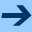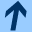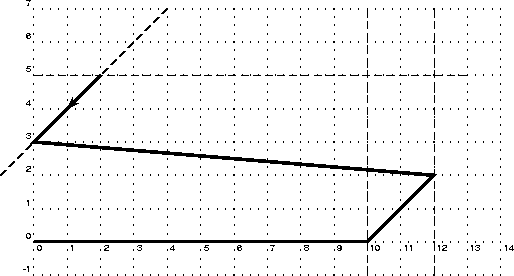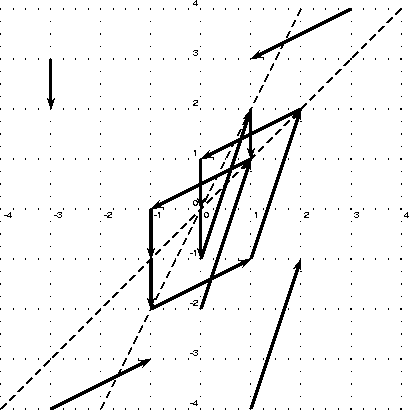Small examples in NBac format

## Small examples in NBac format

You can easily modify the properties contained in these examples. The property is defined by the state variable ok.

incalt
This is the running example of the tutorial. We give variations of it, using as parameters the number of Boolean and numerical variables. p numerical variables are incremented one after the other, while the control moves according to a ring of size 2n defined by n Boolean variables. The goal is to check the invariance property x0 >= x1 >= x2... where the xi are the numerical variables, xi being incremented before xi+1. The naming scheme is incalt-P-N_xyz.ba; P and N have already been described, and xyz means that the property to be checked is x >= y >= z (i.e., we do not necessarily check the general property on all the numerical variables). This example shows how the tool unfold the implicit control structure in order to prove the property.
plane
These are 2 small, purely numerical examples, in 2 dimensions, that shows how the tool can split locations according to numerical constraints. The trajectory of plane1.ba is:And the trajectory of plane2.ba isBertrand Jeannet, February 10, 2011Small examples in NBac format